## 预习报告

### 内容一

#### JK 触发器功能/真值表

CP 时钟JK工作状态$Q^+$
$\downarrow$00保持Q
$\downarrow$01置零0
$\downarrow$10置一1
$\downarrow$11翻转$\overline Q$

#### 用 Proteus 设计电路，并运行仿真

$0000\to1111\to1110\to1101\to1100\to1011\to1010\to1001\to1000\to0111\to0110\to0101\to0100\to0011\to0010\to0001\to0000\to\dots$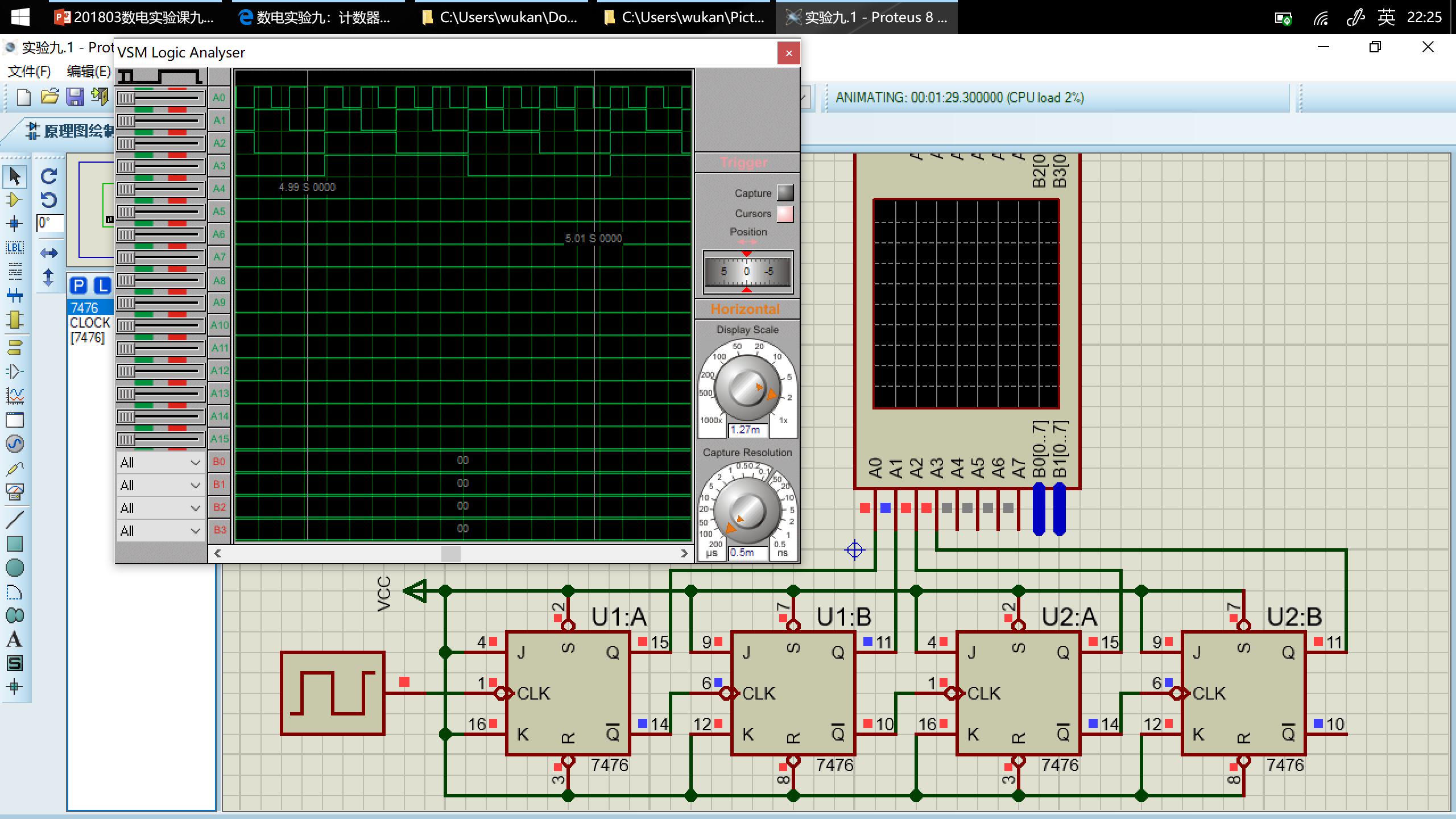### 内容二

#### 用 Proteus 设计电路，并运行仿真

$0000\to0001\to0010\to0011\to0100\to0101\to0110\to0111\to1000\to1001\to1010\to1011\to1100\to1101\to1110\to1111\to0000\dots$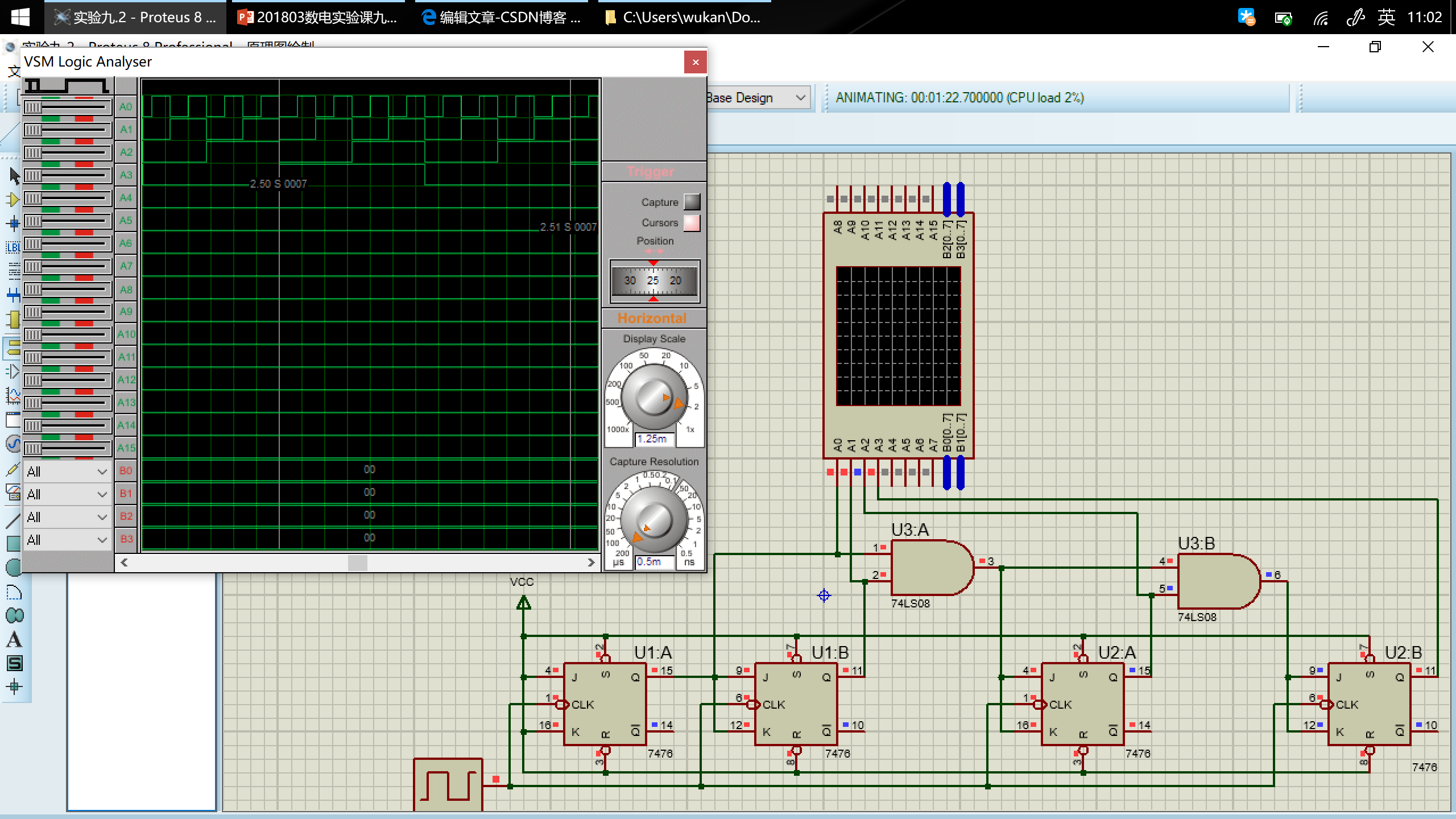### 内容三

#### 74LS194 功能/真值表

CP 时钟$\overline{Cr}$清零${S_1}$${S_0}工作状态Q_A^+$$Q_B^+$$Q_C^+$$Q_D^+$
$\uparrow$0XX置零0000
$\uparrow$100保持$Q_A$$Q_B$$Q_C$$Q_D \uparrow101右移D_{SR}$$Q_A$$Q_B$$Q_C$
$\uparrow$110左移$Q_B$$Q_C$$Q_D$$D_{SL} \uparrow111并行送数D_0$$D_1$$D_2$$D_3$

#### 用 Proteus 设计电路，并运行仿真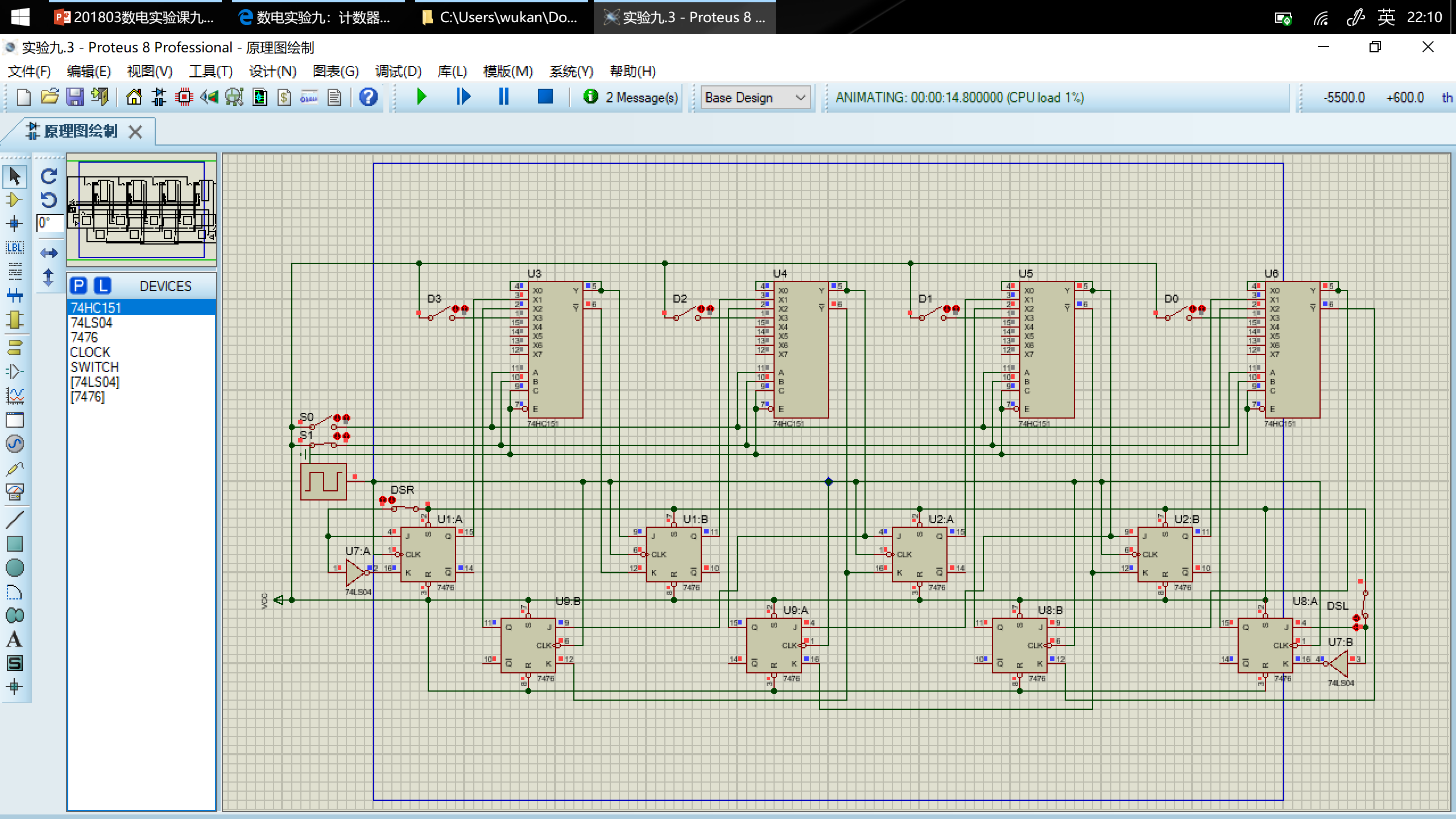### 内容四

$0001\to0010\to0011\to0100\to0101\to0110\to0111\to1000\to1001\to1010\to1011\to1100\to0001\to\dots$

#### 次态卡诺图

$Q_3^+Q_2^+\setminus Q_1^+Q_0^+$00011110
00xxxx001001000011
010101011010000111
110001xxxxxxxxxxxx
101001101011001011

#### 卡诺图化简得到每个触发器方程

$Q_3^+Q_2^+\setminus Q_1^+Q_0^+$00011110
00x000
010010
110xxx
101111

$Q_3^+=Q_3\overline{Q_2}+\overline{Q_3}Q_2Q_1Q_0$

$Q_3^+Q_2^+\setminus Q_1^+Q_0^+$00011110
00x010
011101
110xxx
100010

$Q_2^+=\overline{Q_3}Q_2\overline{Q_1}+\overline{Q_2}Q_1Q_0+\overline{Q_3}Q_2\overline{Q_0}$

$Q_3^+Q_2^+\setminus Q_1^+Q_0^+$00011110
00x101
010101
110xxx
100101

$Q_1^+=Q_1\overline{Q_0}+\overline{Q_1}Q_0$

$Q_3^+Q_2^+\setminus Q_1^+Q_0^+$00011110
00x001
011001
111xxx
101001

$Q_0^+=\overline{Q_0}$

#### 驱动器方程

$J_3=Q_2Q_1Q_0,K_3=Q_2$

$J_2=Q_1Q_0,K_2=\overline{\overline{Q_3}(\overline{Q_1}+\overline{Q_0})}=Q_3+Q_1Q_0$

$J_1=K_1=Q_0$

$J_0=K_0=1$

#### 用 Proteus 设计电路，并运行仿真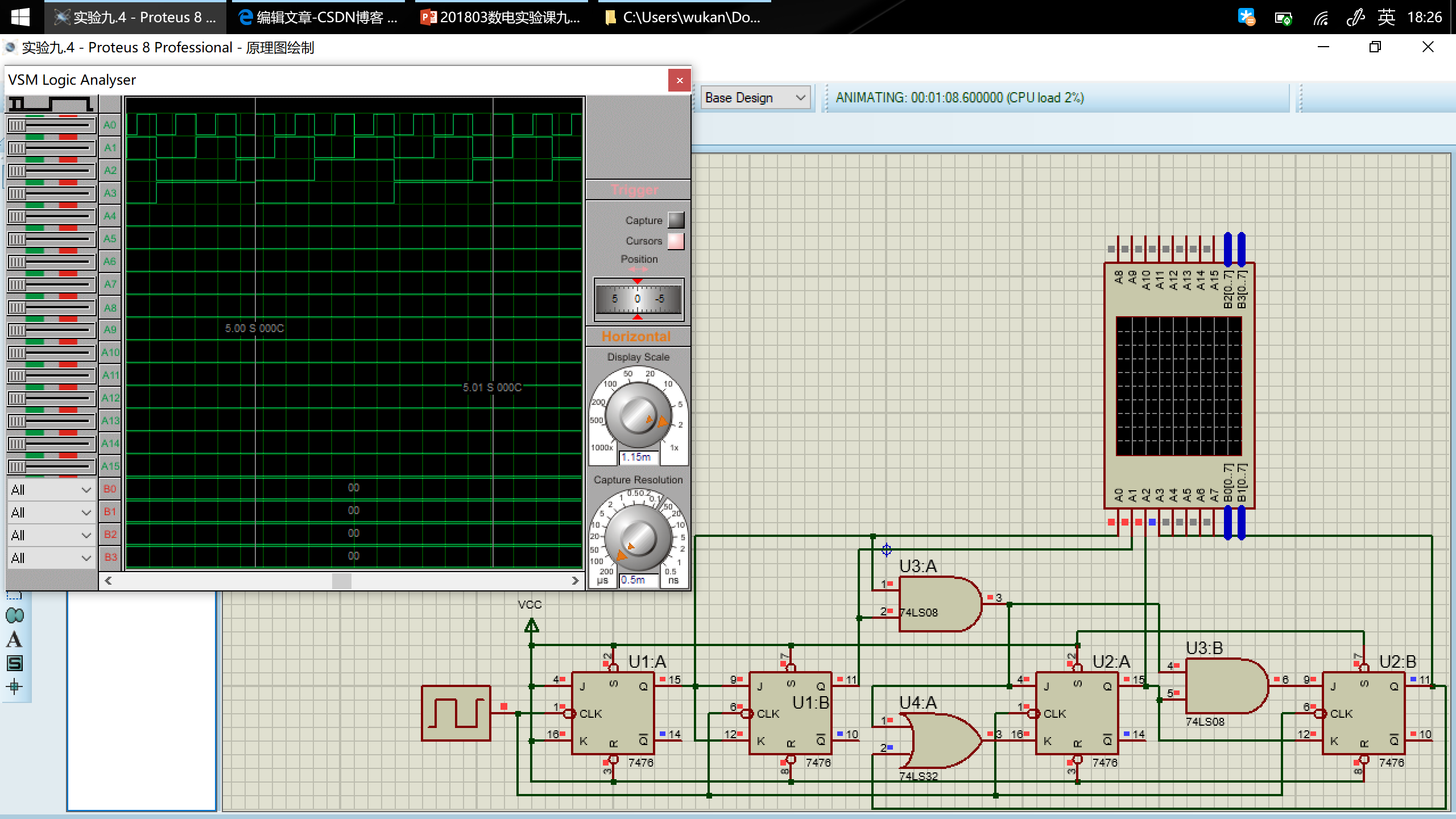### 内容五

#### 次态卡诺图

$Q_3^+Q_2^+\setminus Q_1^+Q_0^+$00011110
00xxxx110000100001
010011010001100101
111011xxxxxxxxxxxx
100111100010101001

#### 卡诺图化简得到每个触发器方程

$Q_3^+Q_2^+\setminus Q_1^+Q_0^+$00011110
00x100
010000
111xxx
100111

$Q_3^+=Q_3Q_2+Q_3Q_1+Q_3Q_0+\overline{Q_3}\,\overline{Q_2}\,\overline{Q_1}$

$Q_3^+Q_2^+\setminus Q_1^+Q_0^+$00011110
00x100
010111
110xxx
101000

$Q_2^+=\overline{Q_3}\,\overline{Q_2}\,\overline{Q_1}+\overline{Q_2}\,\overline{Q_1}\,\overline{Q_0}+Q_2Q_1+Q_2Q_0$

$Q_3^+Q_2^+\setminus Q_1^+Q_0^+$00011110
00x010
011010
111xxx
101010

$Q_1^+=Q_1Q_0+\overline{Q_1}\,\overline{Q_0}$

$Q_3^+Q_2^+\setminus Q_1^+Q_0^+$00011110
00x001
011001
111xxx
101001

$Q_0^+=\overline{Q_0}$

#### 驱动方程

$J_3=\overline{Q_2}\,\overline{Q_1},K_3=\overline{Q_2+Q_1+Q_0}=\overline{Q_2}\,\overline{Q_1}\,\overline{Q_0}$

$J_2=\overline{Q_1}\,\overline{Q_3}+\overline{Q_1}\,\overline{Q_0},K_2=\overline{Q_1+Q_0}=\overline{Q_1}\,\overline{Q_0}$

$J_1=K_1=\overline{Q_0}$

$J_0=K_0=1$

#### 用 Proteus 设计电路，并运行仿真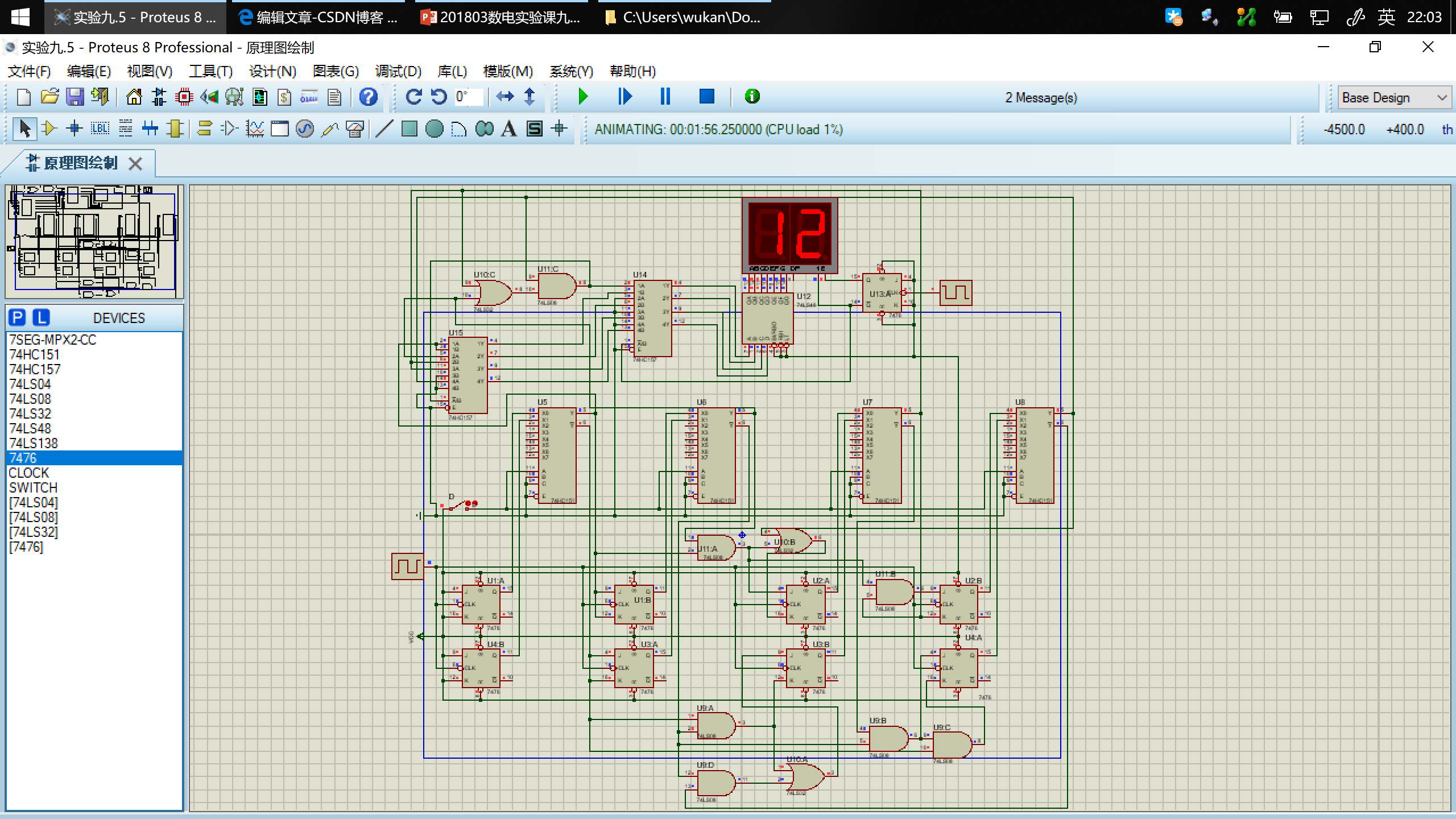#### 用 Vivado 设计电路，并烧写到 Basys3 实验板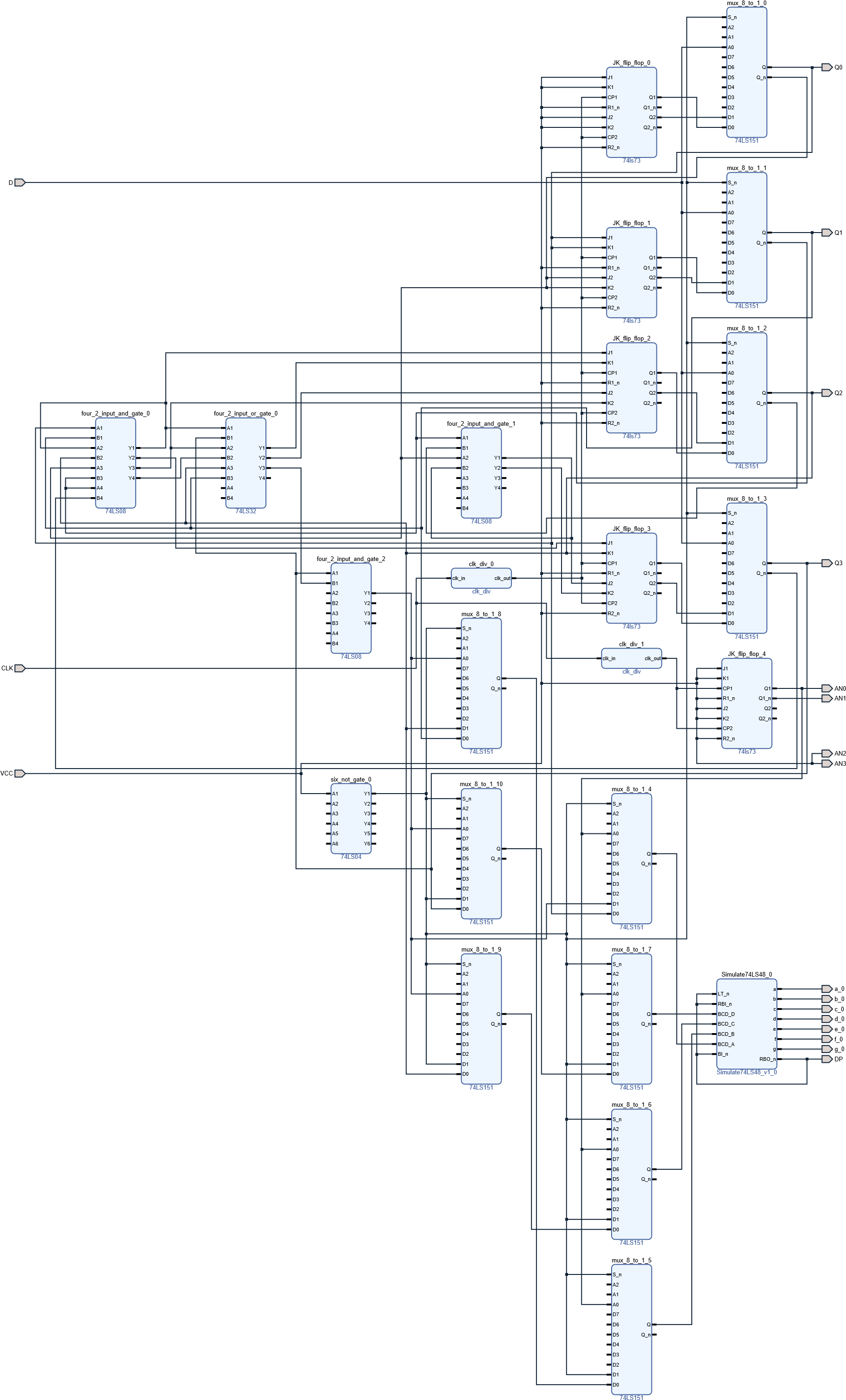##### 端口映射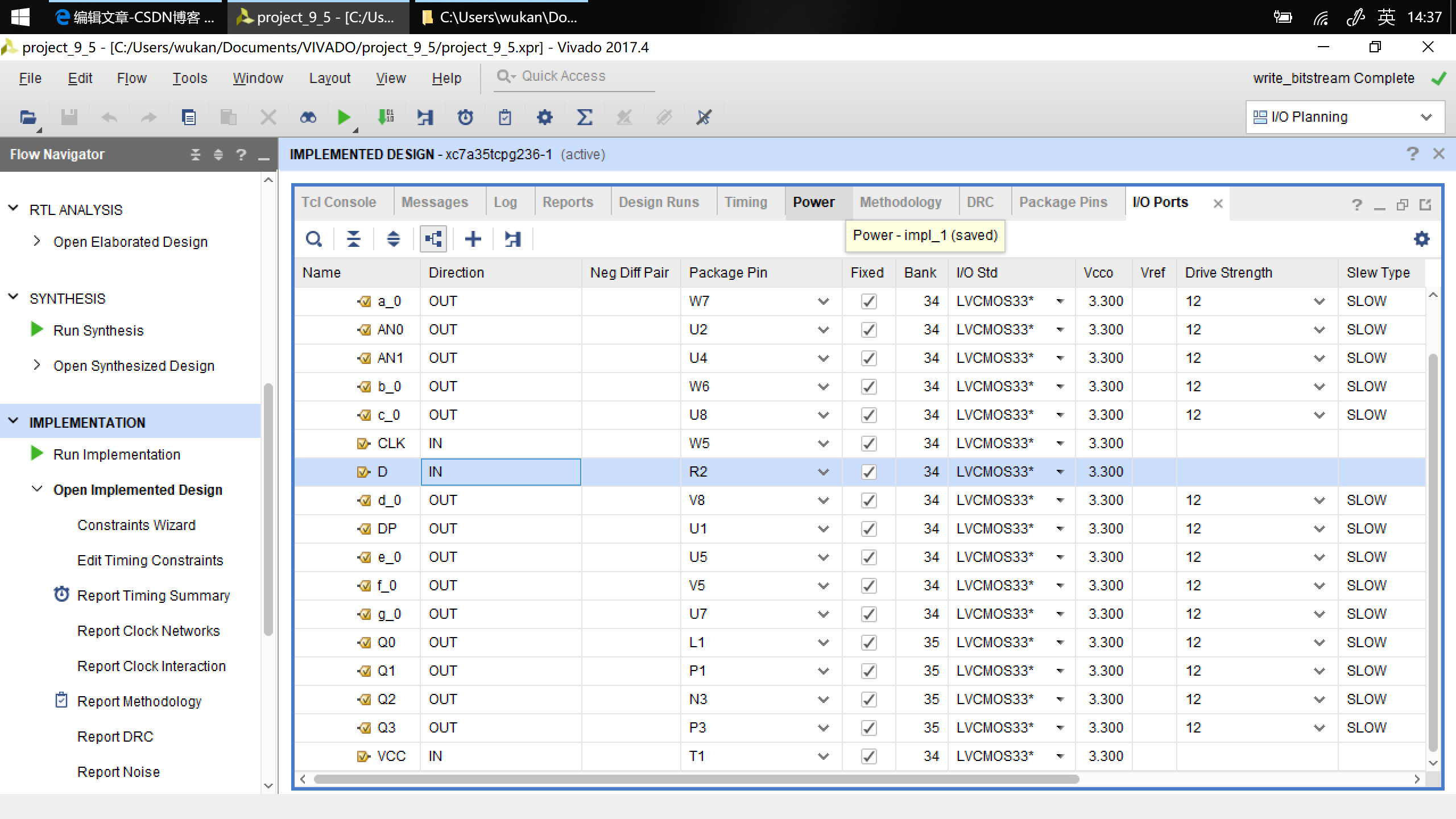##### 烧写到 Basys3 实验板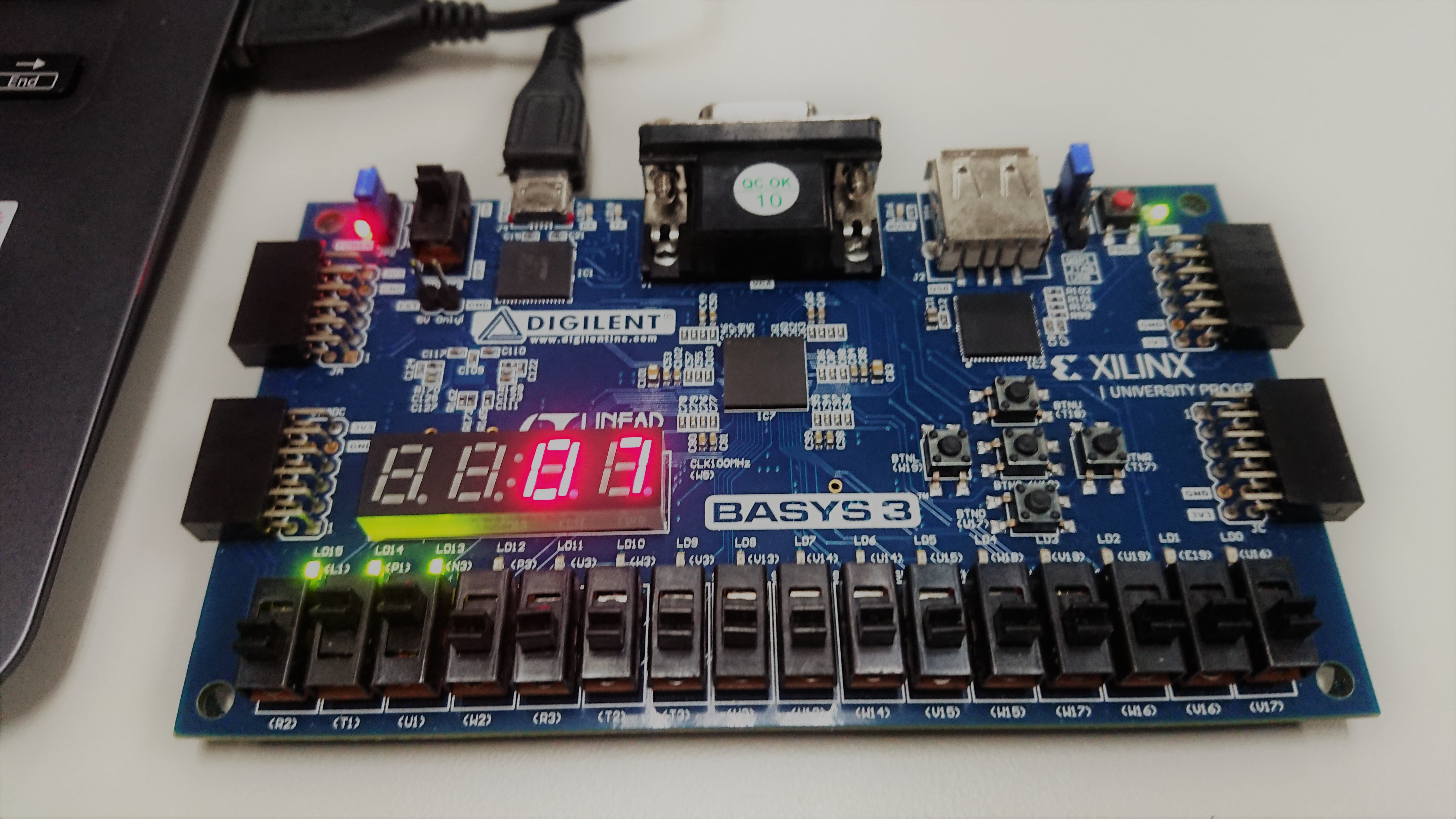## 实验报告

### 内容四

#### 转换电路的效果检验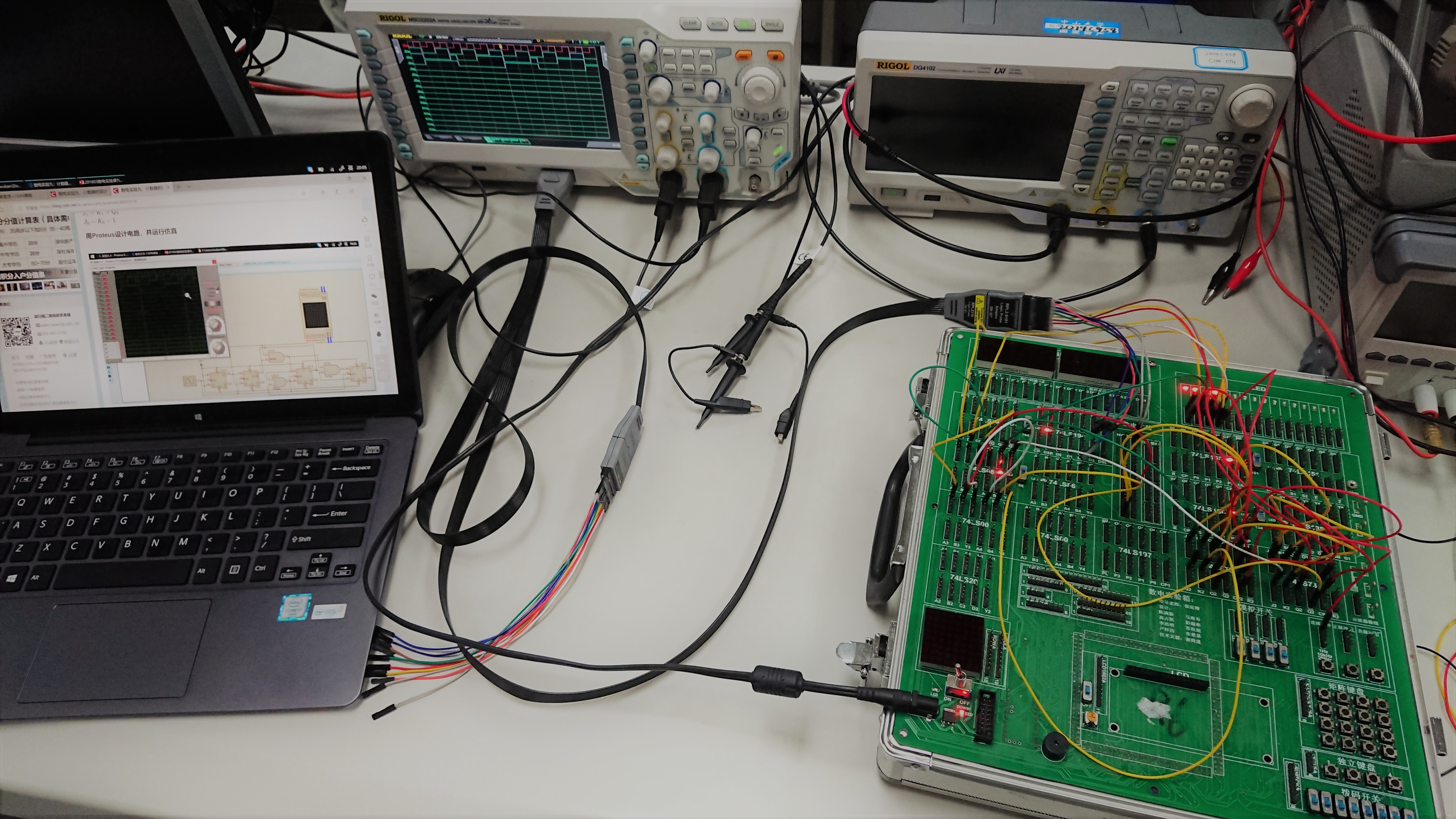#### 波形分析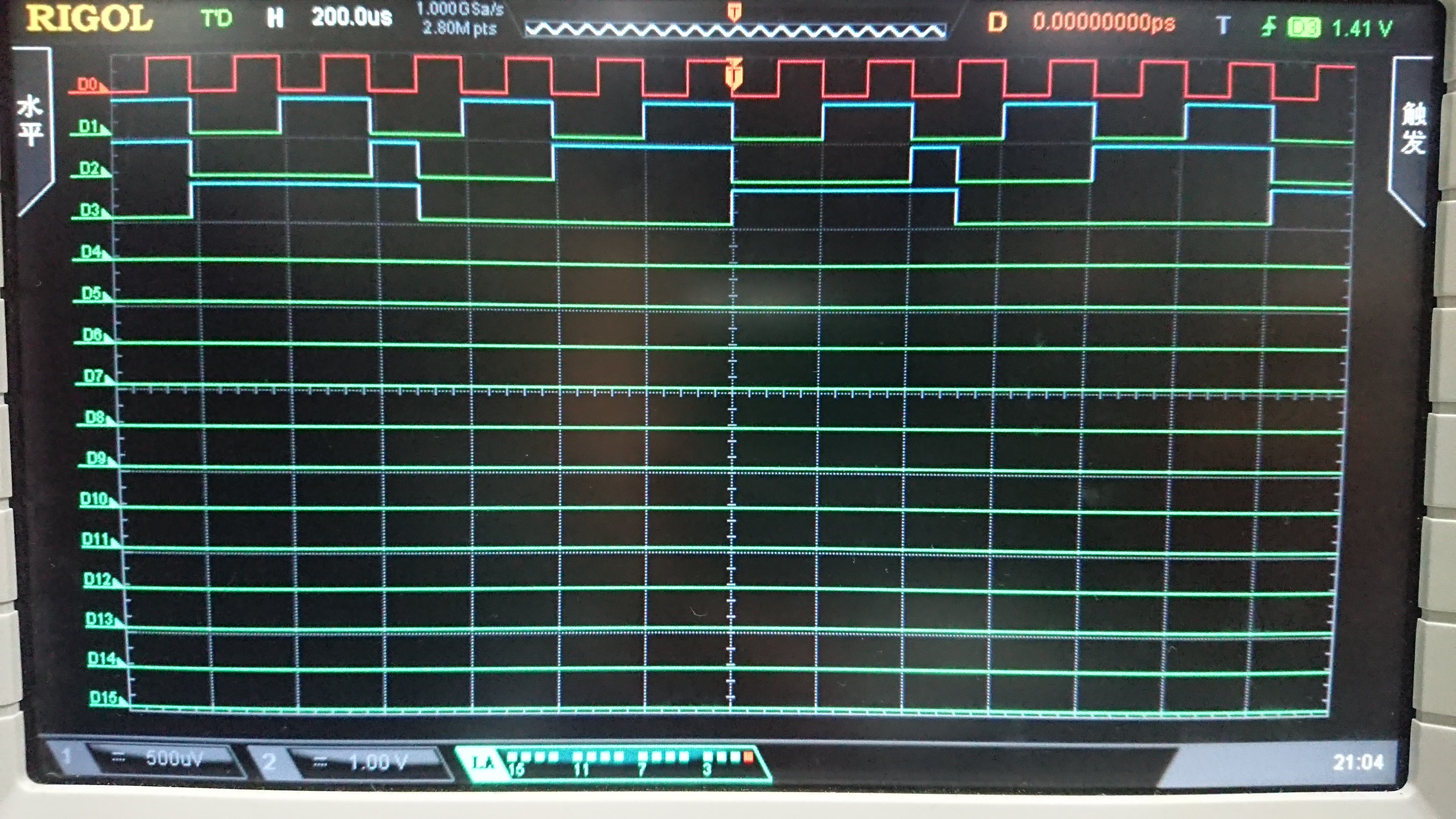### 实验心得和体会

1. 通过本次实验，我了解了 portuse 仿真软件上开关的使用。
2. 通过本次实验，我熟悉了时序逻辑电路的分析步骤和方法。
3. 通过本次实验，我更熟悉了示波器的使用。
4. 通过本次实验，我提高了对错误电路的调试能力。
5. 通过本次实验，我提高了电路的推导和检查能力。